# In a chemical reaction, exactly 2 mol of substance A react to produce exactly 3 mol...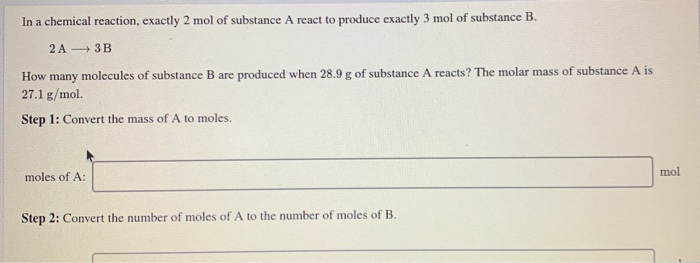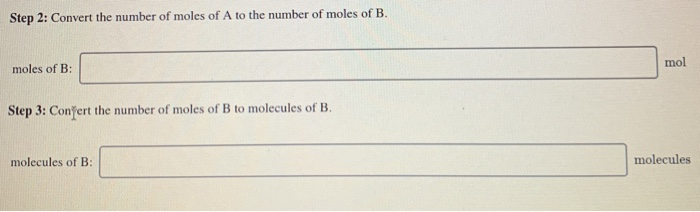In a chemical reaction, exactly 2 mol of substance A react to produce exactly 3 mol of substance B. 2A-3B How many molecules of substance B are produced when 28.9 g of substance A reacts? The molar mass of substance A is 27.1 g/mol Step 1: Convert the mass of A to moles. moles of A: mol Step 2: Convert the number of moles of A to the number of moles of B.
Step 2: Convert the number of moles of A to the number of moles of B. moles of B: mol Step 3: Conğert the number of moles of B to molecules of B. molecules of B: molecules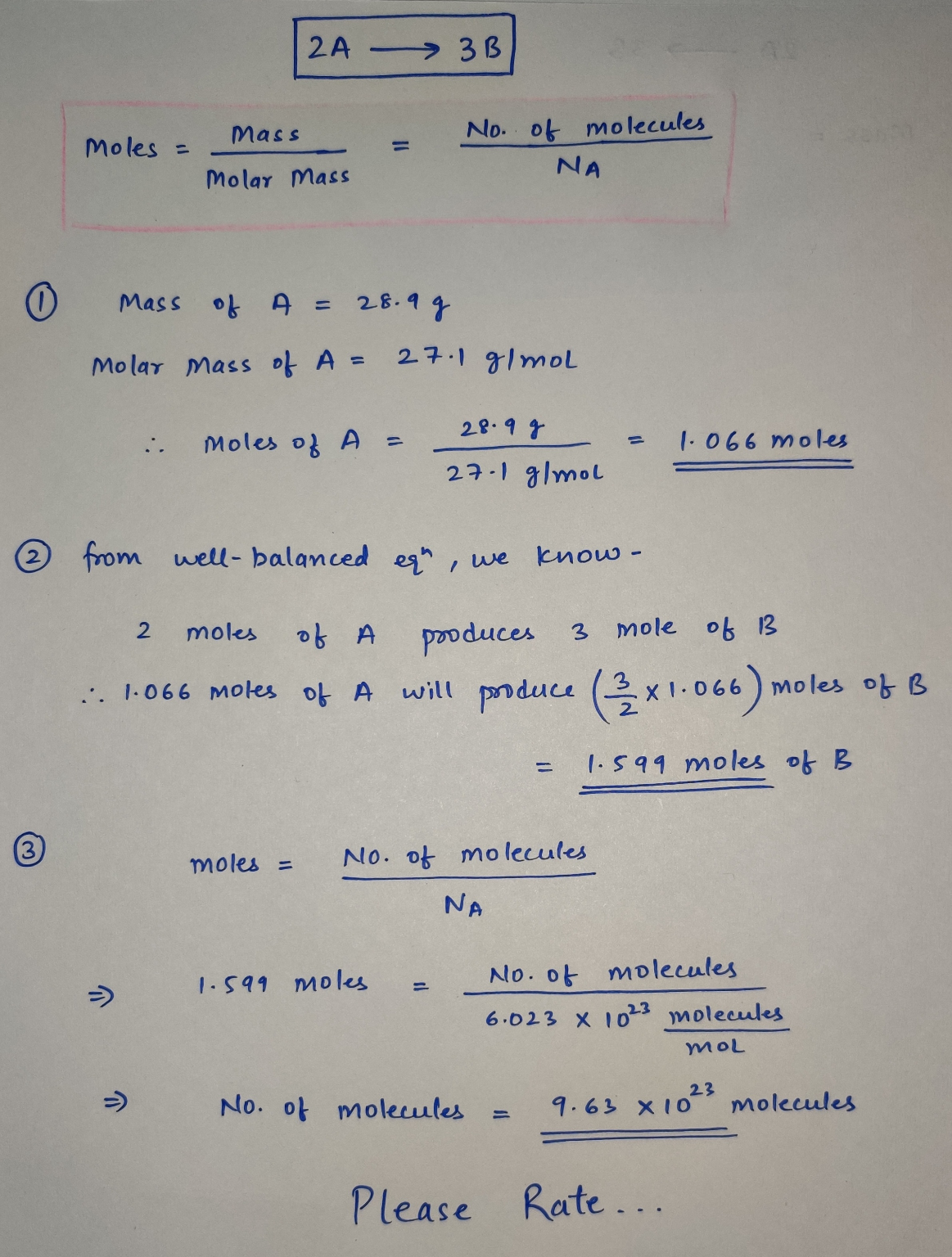#### Earn Coin

Coins can be redeemed for fabulous gifts.

Similar Homework Help Questions
• ### In a chemical reaction, exactly 2 mol of substance A react to produce exactly 3 mol...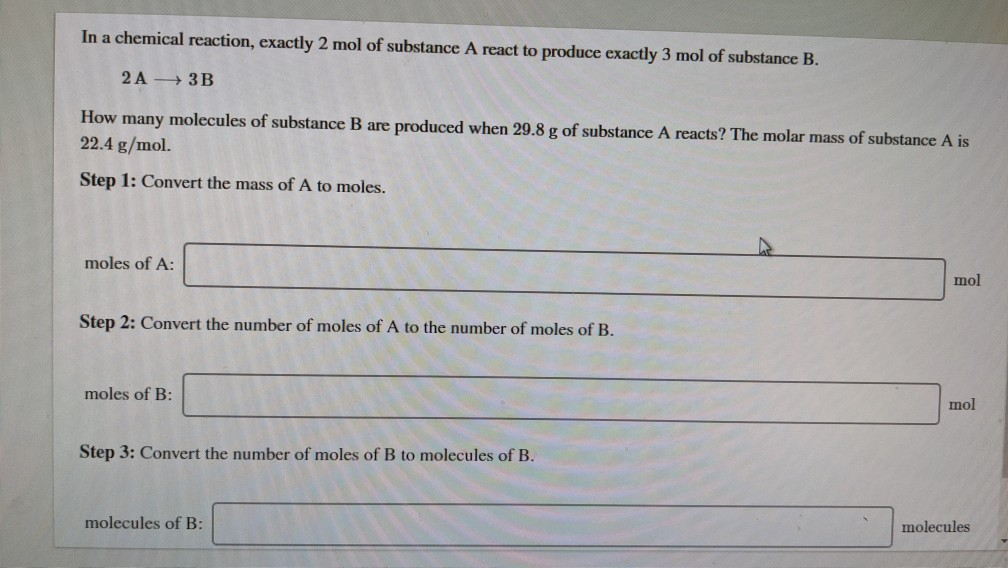In a chemical reaction, exactly 2 mol of substance A react to produce exactly 3 mol of substance B. 2 A 3B How many molecules of substance B are produced when 29.8 g of substance A reacts? The molar mass of substance A is 22.4 g/mol. Step 1: Convert the mass of A to moles. moles of A: mol Step 2: Convert the number of moles of A to the number of moles of B. moles of B: mol Step...

• ### In a chemical reaction, exactly 2 mol of substance A react to produce exactly 3 mol...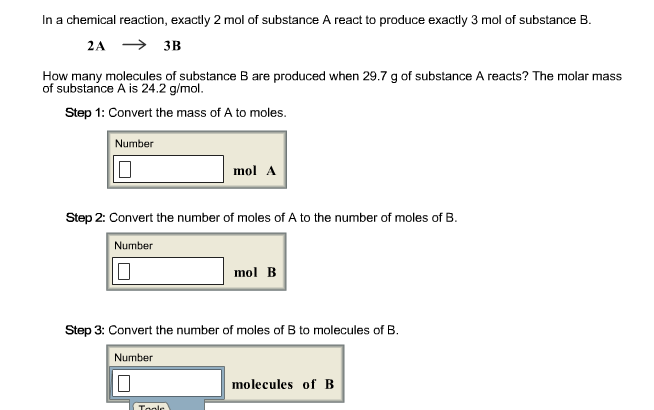In a chemical reaction, exactly 2 mol of substance A react to produce exactly 3 mol of substance B. How many molecules of substance B are produced when 29.7 g of substance A reacts? The molar mass of substance A is 24.2 g/mol. Convert the mass of A to moles. Convert the number of moles of A to the number of moles of B. Convert the number of moles of B to molecules of B.

• ### In a chemical reaction, exactly 2 mol of substance A reacts to produce exactly 3 mol...In a chemical reaction, exactly 2 mol of substance A reacts to produce exactly 3 mol of substance B. 2A rightarrow 3 B How many molecules of substance B are produced when g of substance A reacts? The molar mass of substance A is 19.1 g/mol Convert the mass of A to moles Convert the number of moles of A to the number of mole of B. Convert the number of moles of B to molecules of B.

• ### 121 - Fall19 - PIKUL > Activities and Due Dates > Ch 5 HW Assignment Score:...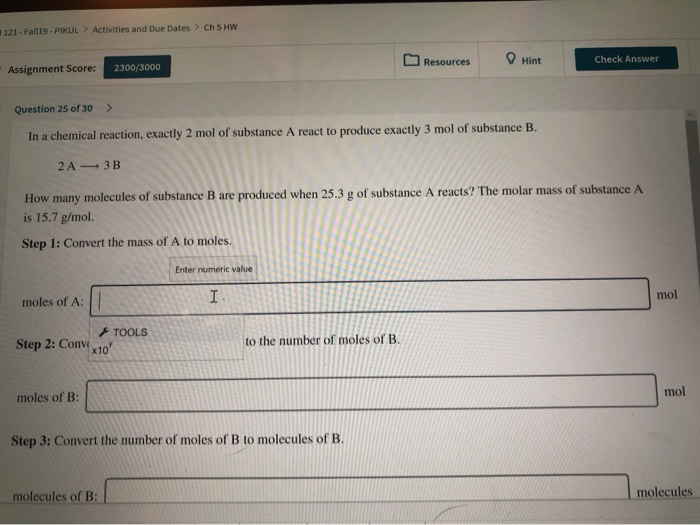121 - Fall19 - PIKUL > Activities and Due Dates > Ch 5 HW Assignment Score: 2300/3000 Resources Hin Check Answer Question 25 of 30 > In a chemical reaction, exactly 2 mol of substance A react to produce exactly 3 mol of substance B. 2A - 3B How many molecules of substance B are produced when 25.3 g of substance A reacts? The molar mass of substance A is 15.7 g/mol Step 1: Convert the mass of A to...

• ### Assignment Score: 375/800 Resources O Hint Check Answer < Question 8 of 8 > In a...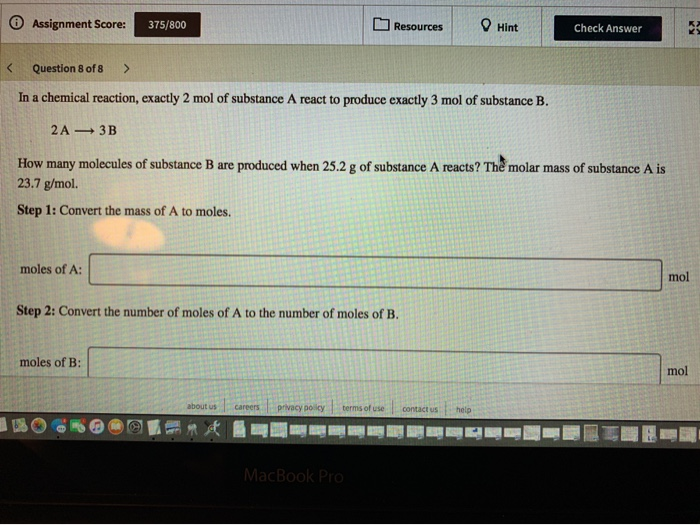Assignment Score: 375/800 Resources O Hint Check Answer < Question 8 of 8 > In a chemical reaction, exactly 2 mol of substance A react to produce exactly 3 mol of substance B. 2A — 3B How many molecules of substance B are produced when 25.2 g of substance A reacts? The molar mass of substance A is 23.7 g/mol. Step 1: Convert the mass of A to moles. moles of A: Step 2: Convert the number of moles of...

• ### In the following chemical reaction, 2 mol of A will react with 1 mol of B...

In the following chemical reaction, 2 mol of A will react with 1 mol of B to produce 1 mol of A2B without anything left over: 2A+B→A2B But what if you're given 2.8 mol of A and 3.2 mol of B? The amount of product formed is limited by the reactant that runs out first, called the limiting reactant. To identify the limiting reactant, calculate the amount of product formed from each amount of reactant separately: 2.8 mol A×1 mol...

• ### A Review Constants Periodic Table How many grams of NH3 can be produced from 4.74 mol...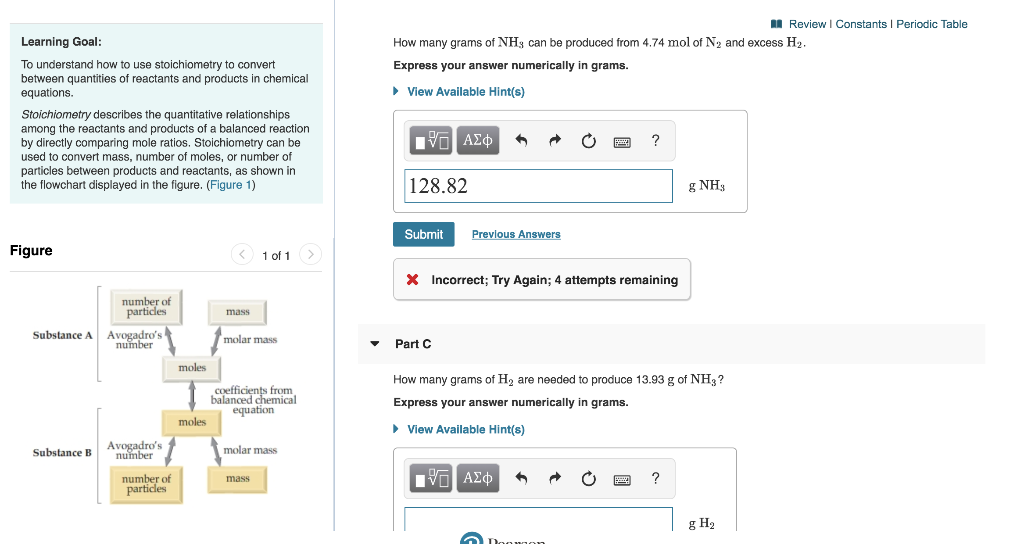A Review Constants Periodic Table How many grams of NH3 can be produced from 4.74 mol of Ny and excess H2, Express your answer numerically in grams. View Available Hint(s) Learning Goal: To understand how to use stoichiometry to convert between quantities of reactants and products in chemical equations. Stoichiometry describes the quantitative relationships among the reactants and products of a balanced reaction by directly comparing mole ratios. Stoichiometry can be used to convert mass, number of moles, or number...

• ### Stoichiometry (How are masses of reactants and products related?) Model 1: The Balanced Chemical Equation As...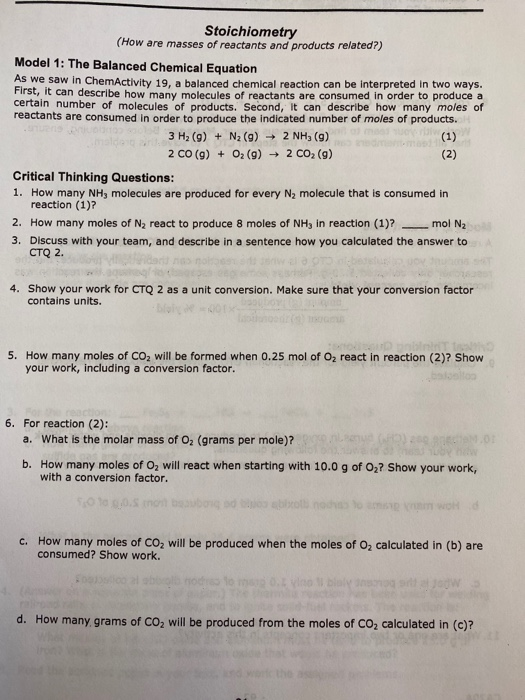Stoichiometry (How are masses of reactants and products related?) Model 1: The Balanced Chemical Equation As we saw in ChemActivity 19. a balanced chemical reaction can be interpreted in two ways. First, it can describe how many molecules of reactants are consumed in order to produce a certain number of molecules of products. Second, it can describe how many moles of reactants are consumed in order to produce the indicated number of moles of products. | 3 H2 (9) +...

• ### AP Chemistry Number of Moles Practice: Part A) Lithium and nitrogen react to produce lithium nitride:6Li(s)+N2(g)→2Li3N(s)....

AP Chemistry Number of Moles Practice: Part A) Lithium and nitrogen react to produce lithium nitride:6Li(s)+N2(g)→2Li3N(s). How many moles of lithium nitride are produced when 0.630 mol of lithium react in this fashion?    Part B) How many moles of BCl3 are needed to produce 10.0 g of HCl(aq) in the following reaction? BCl3(g) + 3 H2O(l) → 3 HCl(aq) + B(OH)3(aq) Part C) How many moles of nitrogen are formed when 58.6 g of KNO3 decomposes according to the...

• ### Date: Period: Name: You Try... 4. Given the following chemical equation 4P + 50; → 2...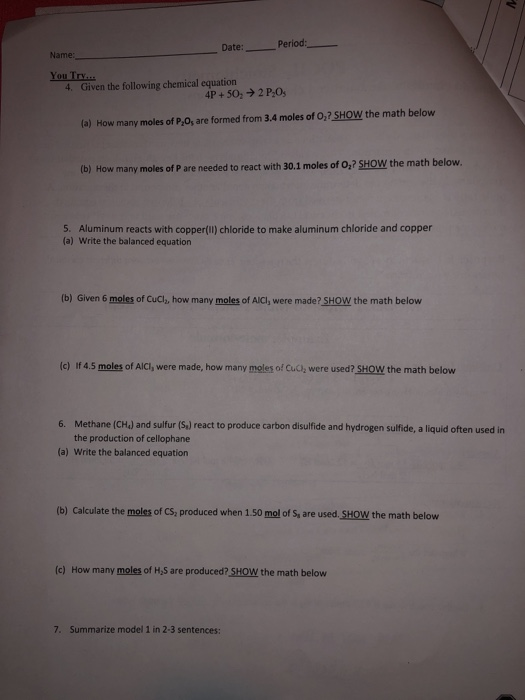Date: Period: Name: You Try... 4. Given the following chemical equation 4P + 50; → 2 PO (a) How many moles of P,0, are formed from 3.4 moles of O,? SHOW the math below (b) How many moles of P are needed to react with 30,1 moles of O,? SHOW the math below. 5. Aluminum reacts with copper(ll) chloride to make aluminum chloride and copper (a) Write the balanced equation (b) Given 6 moles of Cuch, how many moles of...